# Gyroscopic exercise tool facts for kids

Kids Encyclopedia Facts

A gyroscopic exercise tool is a device used to exercise the wrist as part of physical therapy or in order to build palm, forearm and finger strength. It can also be used as a unique demonstration of some aspects of rotational dynamics. The device consists of a tennis ball-sized plastic or metal shell around a free-spinning mass, which is started with a short rip string. Once the gyroscope inside is going fast enough, a person holding the device can accelerate the spinning mass to high revolution rates by moving the wrist in a circular motion.

## Mechanics

The device consists of a spinning mass inside an outer shell. The shell almost completely covers the mass inside, with only a small round opening allowing the gyroscope to be manually started. The spinning mass is fixed to a thin metal axle, each end of which is trapped in a circular, equatorial groove in the outer shell. A lightweight ring with two notches in it for the ends of the axle rests in the groove. This ring can slip in the groove; it holds the spinning gyroscope centered in the shell, preventing the two from coming into contact (which would slow the gyro down), but still allowing the orientation of the axle to change.

Since the spinning mass is balanced, the only possibility to speed up the rotation is for the sides of the groove to exert forces on the ends of the axle. Furthermore, the normal and axial forces will have no effect, so tangential force must be provided by friction. If the axle is stationary, the friction will only act to slow down the rotation, but the situation is very different if the axle is turned by applying a torque.

This can be accomplished by tilting the shell in any direction except in the plane of the groove or around an axis aligned with the axle, and results in a shift of the axle ends along the groove. The direction and speed of the shift can be found from the formula for the precession of a gyroscope: the applied torque is equal to the cross product of the angular velocity of precession and the angular momentum of the spinning mass. The most important observation here is that the direction is such that, if the torque is large enough, the friction between the axle and the surface of the groove will speed up the rotation.

This may seem odd. After all, if the axle were shifting in a horizontal groove, the friction on one end that acts to speed up the rotation would be canceled by the friction at the other end, operating in the opposite direction. The difference is that a torque is being applied, so one end of the axle is pushing against one side of the groove, while the other end is pushing against the other side. Likewise, it does not matter in which direction the torque is applied. If the torque is reversed, each end of the axle will then be pressing against the opposite side of the groove, but the direction of precession is also reversed. The only restriction is that the relative speed of the surface of the axle and the side of the groove due to precession,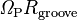$\mathit{\Omega}_{\mathrm{P}} R_{\mathrm{groove}}$, must exceed the relative speed due to the rotation of the spinning mass,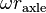$\omega r_{\mathrm{axle}}$. The minimum torque required to meet this condition is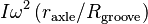$I \omega^2 \left( r_{\mathrm{axle}} / R_{\mathrm{groove}} \right)$, where I is the moment of inertia of the spinning mass, and ω is its angular velocity.

Since an acceleration of the rotation will occur regardless of the direction of the applied torque, as long as it is large enough, the device will function without any fine-tuning of the driving motion. The tilting of the shell does not have to have a particular phase relationship with the precession or even to have the same frequency. Since sliding (kinetic) friction is usually nearly as strong as static (sticking) friction, it is also not necessary to apply precisely the value of torque which will result in the axle rolling without slipping along the side of the groove. These factors allow beginners to learn to speed up the rotation after only a few minutes of practice.

By applying the proportionality of the force of friction to the normal force,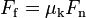$F_\mathrm{f} = \mu_\mathrm{k} F_\mathrm{n}$, where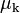$\mu_\mathrm{k}$ is the kinetic coefficient of friction, it can be shown that the torque spinning up the mass is a factor of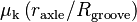$\mu_\mathrm{k} \left( r_{\mathrm{axle}} / R_{\mathrm{groove}} \right)$ smaller than the torque applied to the shell. Since frictional force is essential for the device's operation, the groove must not be lubricated.

As explained, the energy supplied by the external momentum (the hand and arm muscles) can not be directly converted into the rotational energy of the gyroscope around its own axis, but it is converted into the energy of precession rotation. Due to the friction between the gyroscope shaft and the sides of the groove, part of this energy is converted into energy of rotation around the gyroscope's own axis accelerating it. This happens when there is friction and a round object, like a bowling ball, is thrown along a horizontal surface. Part of the ball's kinetic energy is converted to rotational energy due to friction.Gyroscopic exercise tool Facts for Kids. Kiddle Encyclopedia.